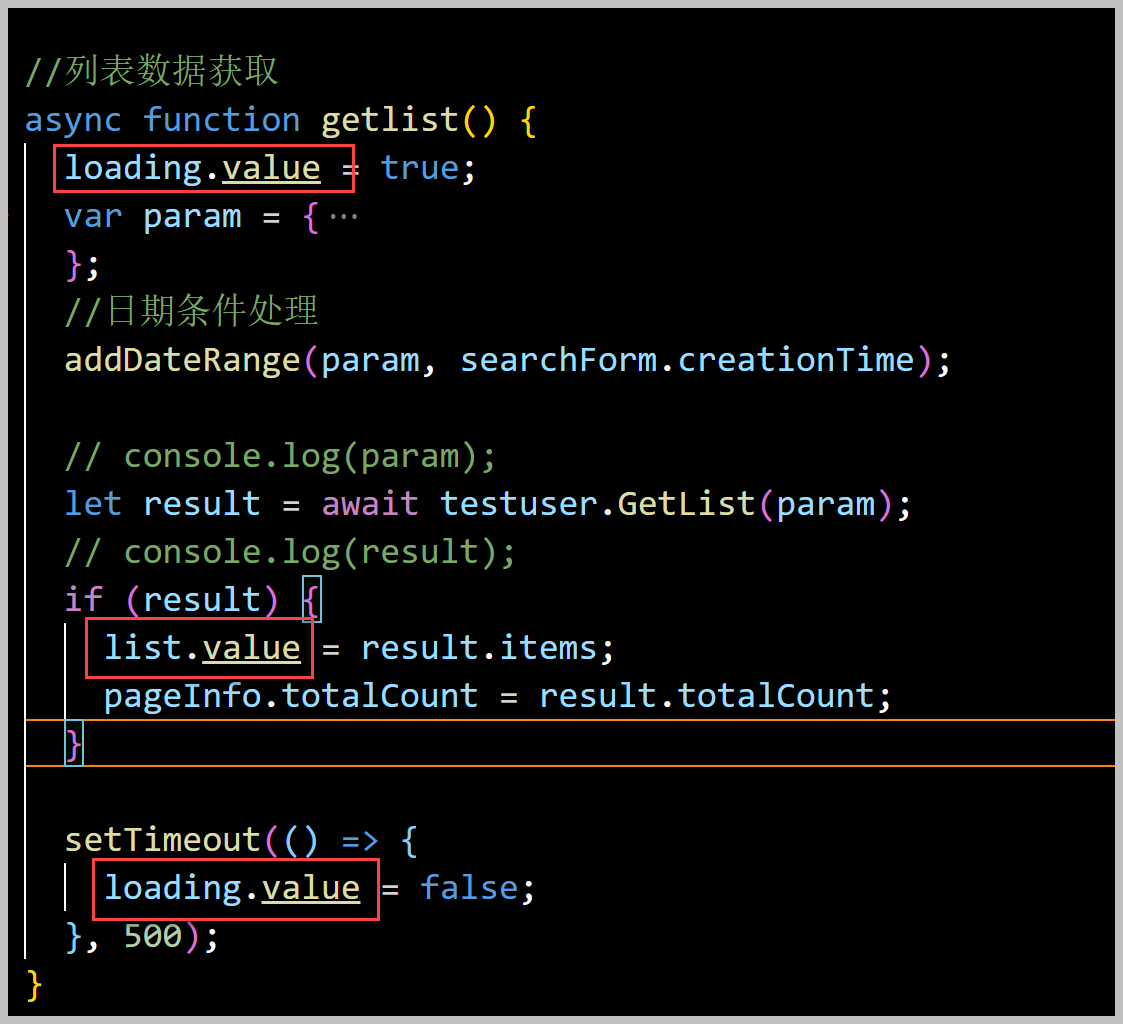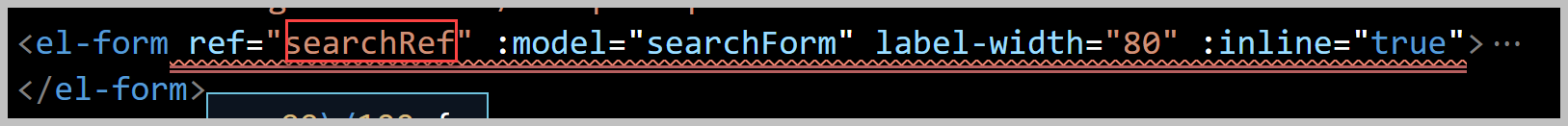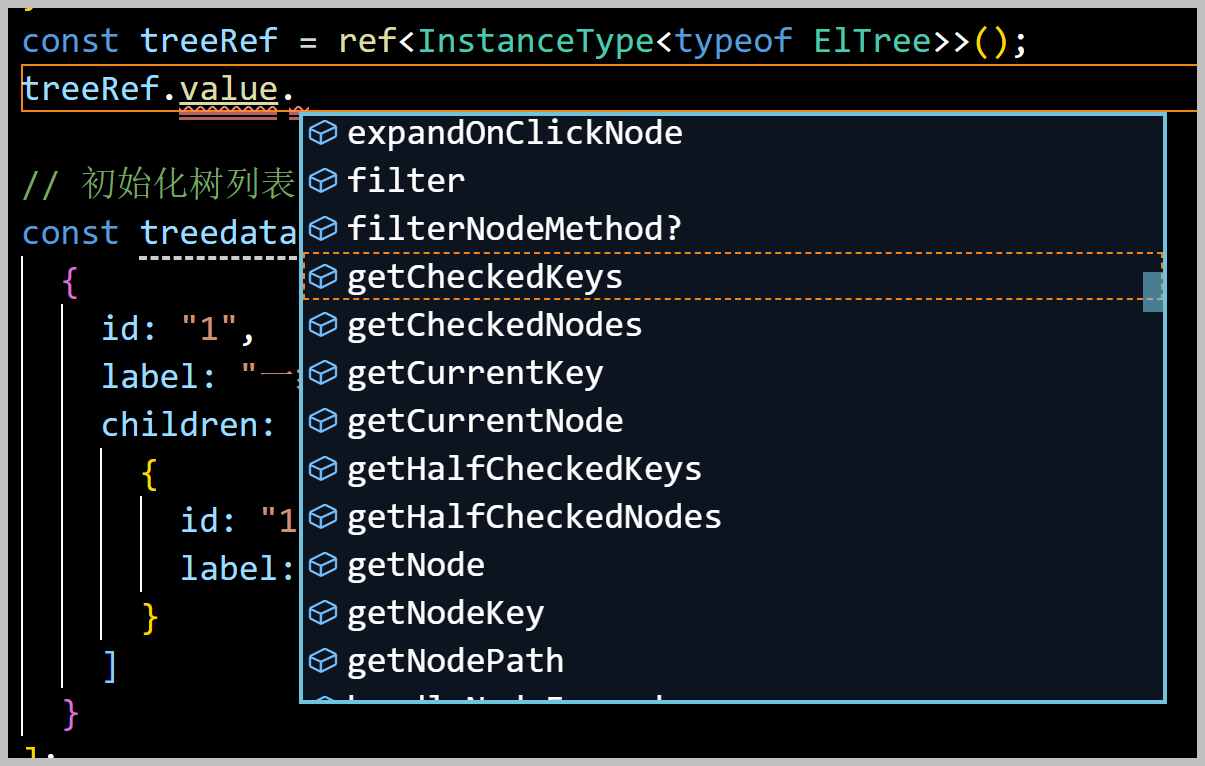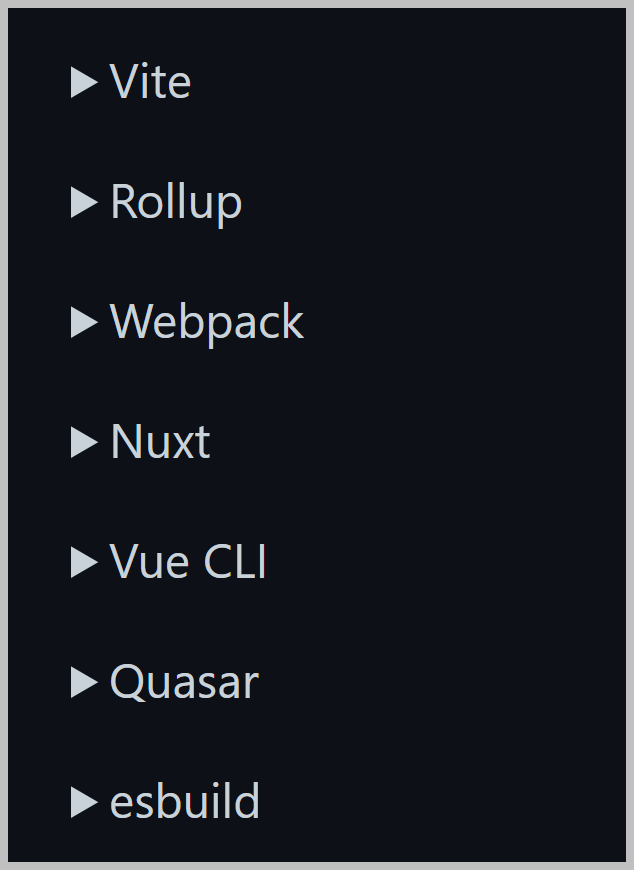## 基于SqlSugar的开发框架循序渐进介绍（11）-- 使用TypeScript和Vue3的Setup语法糖编写页面和组件的总结

TypeScript（简称ts）是微软推出的静态类型的语言，相比于js，TypeScript拥有强类型、编译器严谨的语法检查、更加严苛的语法，TypeScript 是 JS类型的超集，并支持了泛型、类型、命名空间、枚举等特性，弥补了 JS 在大型应用开发中的不足。TypeScript 是 JavaScript 的强类型版本，最终在浏览器中运行的仍然是 JavaScript，所以 TypeScript 并不依赖于浏览器的支持，也并不会带来兼容性问题。

<script setup lang="ts">  是在单文件组件 (SFC) 中使用组合式 API 的编译时语法糖。script-setup 弱化了vue模板式编程体验，也使得代码更简洁。

### 1、定义组件或者页面名称

<script setup lang="ts">
import { reactive,  ref,  onMounted,  watch,  computed } from "vue";

defineOptions({ name: "MyDictdata" }); //定义组件或页面名称

// 自定义字典控件
import MyDictdata from "./src/my-dictdata.vue";

<my-dictdata v-model="editForm.nationality" type-name="民族" />

### 2、data属性定义

let expandMore = ref(false); //是否展开更多条件
let list = ref([]); // 页面列表数据
let listSelection = ref([]); // 选中记录
let sorting = ref(""); // 排序条件

// 分页条件
let pageInfo = reactive({
pageIndex: 1,
pageSize: 20,
totalCount: 0
});

<!--分页部分 -->
<div class="block" style="height: 70px">
<el-pagination background :current-page="pageInfo.pageIndex" :page-size="pageInfo.pageSize"
:total="pageInfo.totalCount" :page-sizes="[10, 20, 30, 40]" layout="total, sizes, prev, pager, next,jumper"
@size-change="sizeChange" @current-change="currentChange" />
</div>### 3、表单或者组件的ref引用

const searchRef = ref<FormInstance>(); //表单引用

import { FormInstance, FormRules } from "element-plus";<script lang="ts" setup>
import { ref } from 'vue'
import { ElTree } from 'element-plus'

const treeRef = ref<InstanceType<typeof ElTree>>()<script setup lang="ts">
//组件的接口类型
export interface ExposeViewType {
show(id?: string | number): Function;
}

//显示窗口
const show = (id: string | number) => {
if (!isNullOrUnDef(id)) {
testuser.Get(id).then(data => {
Object.assign(viewForm, data);

isVisible.value = true; //显示对话框
});
}
};

//暴露组件属性和方法
defineExpose({
show
});

    <!--查看详细组件界面-->
<view-data ref="viewRef" />

const viewRef = ref<ExposeViewType | null>(); //查看表单引用### 4、组件prop属性定义

prop属性定义，是通过defineProps函数进行处理的，这个defineProps()宏函数支持从它的参数中推导类型，定义的代码如下所示。

<script setup lang="ts">
const props = defineProps<{
foo: string
bar?: number
}>()
</script>

我们也可以将 prop 的类型移入一个单独的接口中：

<script setup lang="ts">
interface Props {
foo: string
bar?: number
}

const props = defineProps<Props>()
</script>

<script setup lang="ts">
import {  reactive,  ref,  onMounted,  watch,  computed} from
vue";

//定义组件名称
defineOptions({ name: "MyDictdata" });

//声明Props的接口类型
interface Props {
placeholder?: string; // 空白提示
typeName?: string; // 字典类型方式，从后端字典接口获取数据
options?: Array<TreeNodeItem>; // 固定列表方式，直接绑定,项目包括id,label属性
modelvalue?: string | number; // 接受外部v-model传入的值
clearable?: boolean; // 是否可以清空
disabled?: boolean; // 是否禁用
multiple?: boolean; // 是否多选
}

//使用默认值定义Props
const props = withDefaults(defineProps<Props>(), {
placeholder: "请选择",
typeName: "",
options: () => {
return [];
},
clearable: true,
disabled: false,
multiple: false,

modelValue: "" //对应自定义控件的v-model的值
});

  <el-form-item label="民族" prop="nationality">
<my-dictdata v-model="editForm.nationality" type-name="民族" />
</el-form-item>

### 5、Emits事件声明

  // 声明事件
const emit = defineEmits(['updateName'])

//声明控件事件
interface Emits {
(e: "update:modelValue", value: string): void;
(e: "change", value: string): void;
}
//定义控件事件
const emit = defineEmits<Emits>();

// 基于类型的声明
const emit = defineEmits<{
(e: 'change', id: number): void
(e: 'update', value: string): void
}>()

function change(data) {
const obj = dictItems.value.find(item => {
return item.id + "" === data;
});
emit("change", obj);
}

  <el-form-item label="状态" prop="state">
<my-dictdata v-model="searchForm.state" :options="Status" @change="change" />
</el-form-item>

### 6、Computed计算函数的使用

「computed」 是Vue中提供的一个计算属性。它被混入到Vue实例中，所有的getter和setter的this上下文自动的绑定为Vue实例。

<script setup lang="ts">
import { computed, ref } from 'vue'

const count = ref(1)

// 通过computed获得doubleCount
const doubleCount = computed(() => {
return count.value * 2
})
// 获取
console.log(doubleCount.value)
</script>

### 7、Watch函数的使用

// 监听外部对props属性变更,如通过ResetFields()方法重置值的时候
watch(
() => props.modelValue,
newValue => {
console.log(newValue);
emit("update:modelValue", newValue + "");
}
);

watch(
() => props.options,
newValue => {
newValue.forEach(item => {
dictItems.value.push(item);
});
}
);

### 8、onMounted函数的使用

//页面初始化加载
onMounted(() => {
getlist();
});

//挂载的时候初始化数据
onMounted(async () => {
var typeName = props.typeName;
var options = props.options;
if (typeName && typeName !== "") {
// 使用字典类型，从服务器请求数据
await dictdata.GetTreeItemByDictType(typeName).then(list => {
if (list) {
list.forEach(item => {
dictItems.value.push({ id: item.id, label: item.label });
});
}
});
} else if (options && options.length > 0) {
// 使用固定字典列表
options.map(item => {
dictItems.value.push({ id: item.id, label: item.label });
});
}

// 设置默认值
keyword.value = props.modelValue;
});

### 9、自定义组件的ModelValue

  <el-form-item label="姓名" prop="name">
<el-input v-model="editForm.name" />
</el-form-item>

  <el-form-item label="出生日期" prop="birthDate">
<el-date-picker v-model="editForm.birthDate" align="right" type="date" placeholder="选择日期"
format="YYYY-MM-DD" />
</el-form-item>

//声明Props的接口类型
interface Props {
modelvalue?: string | number; // 接受外部v-model传入的值
}
//使用默认值定义Props
const props = withDefaults(defineProps<Props>(), {
modelValue: "" //对应自定义控件的v-model的值
});

//声明组件事件
interface Emits {
(e: "update:modelValue", value: string): void;
(e: "change", value: string): void;
}
//定义组件事件
const emit = defineEmits<Emits>();

watch(
() => props.modelValue,
newValue => {
console.log(newValue);
emit("update:modelValue", newValue + "");
}
);

### 10、自定义引入Vue的API和组件

<script setup lang="ts">
import { reactive,  ref,  onMounted,  watch,  computed } from "vue";

const count = ref(0)
const doubled = computed(() => count.value * 2)

npm i -D unplugin-auto-import// vite.config.ts
import AutoImport from 'unplugin-auto-import/vite'

export default defineConfig({
plugins: [
AutoImport({ /* options */ }),
],
})

posted on 2022-07-08 09:45  伍华聪  阅读(2162)  评论(1编辑  收藏  举报